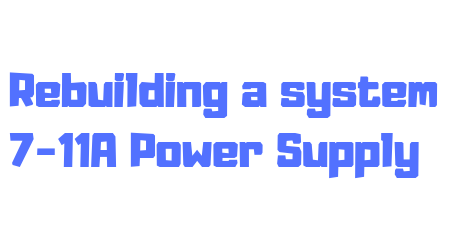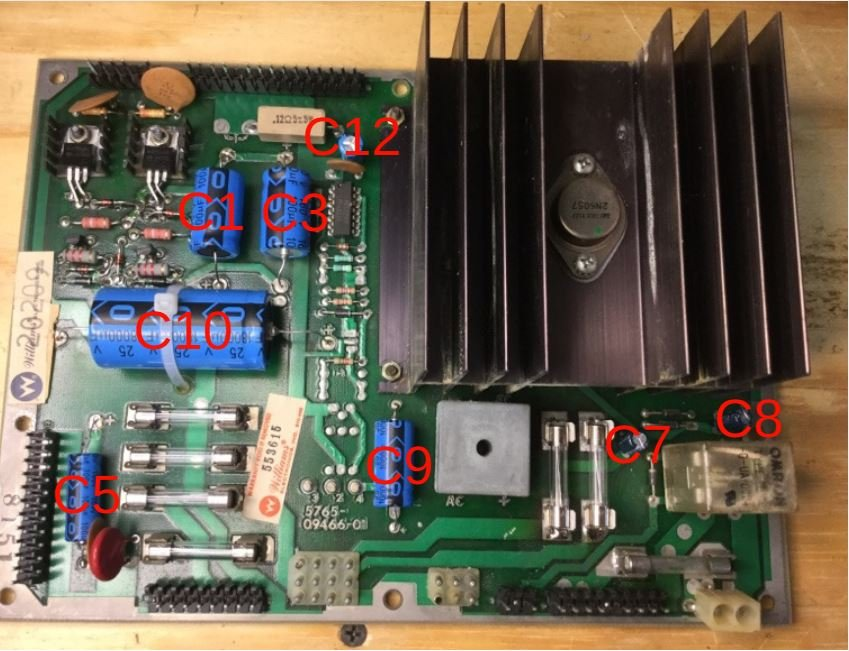System 7-11A Cap Map for Power Supply Rebuild

C1 = 100uf, 150V
C3 = 100uf, 150V
C5 = 100uf, 100V
C9 = 1,000uf, 25V
C10 = 18,000uf, 20V

ZR1 & ZR3 = 1N4730A
ZR2 & ZR4 = 1N4764A
*ZR2 & *ZR4 = 1N4763A (labeled with “*”).
These reduce voltage by 9 volts to 91 volts and help preserve the displays.  If problems with 1N4763As then use 1N7464As listed above.
D3 & D4 = 1N4004
Q1 = MJE15030 (cross legs)**
Q3 = MJE15031 (cross legs)**
Q2 = 2N5401
Q4 = 2N5551
R1 & R4 = 39K ohm, 2 watt
(Orange, White, Orange, Gold)
R2 & R5 = 1.2K ohm, 1 watt
(Brown, Red, Red, Gold)
R3 & R6 = 330K ohm, 1/2 watt
(Orange, Orange, Yellow, Gold)

**later games my not need to cross legs.

Note: Rebuild kits are available in the store for \$14.99 shipped via 1st class.